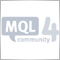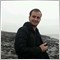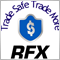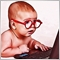# Experts: LotScalp - page 316299

I test the EA now for 4 weeks on a demo EUR/USD of H1 to M1. But he tradet not

TP = 5
SL = 5
lot = 0.01
orders = 1
max OpenTime = 504

my Broker is:
Digits = 5
spread = 1.6-2.2 pips

Which settings one must take?16299

Just started this on a live cent account, will report back on how it performs each week. I'll try to get it up on as well.16299

Does this mean that the leverage in this code is 1:40? thx!3

Hi,

I attached this EA with 4XP 8 digits demo account but no trade is opening while I have not change any inputs. However, back test is ok. means it opens trades.

Kindly help me,

Regards,

Ramsharan978

It's based on the Minimum TP and SL of the server, @ClickPaypal, for the Takeprofit and stop loss better use,

double TP = MarketInfo("USDJPY",MODE_STOPLEVEL)*Point;

double SL = (TP*STOPLOSS)*Point;

extern int STOPLOSS = 10;

in this case when the minimum TP is 4 pips, the SL is 40 pips.

Greetz Wtrader116299

This kills accounts very quickly in multiple 99% backtests I tried on multiple pairs.

-Joe21

Does anyone need an EA to earn 5 pips with a 6000 pips stoploss?41856

A very dangerous Forex robot which is based on not putting an SL point until the open position reaches the desired TP point!310

i can't understand how can it make buy or sell order ?

what's the algorithm ?35

I made some changes to the programs.
Thanks to those who will improve it again.

Historical http://urlz.fr/7q36

```//+------------------------------------------------------------------+
//|                                 LotScalp.mq4 v2 EurUsd 1H        |
//+------------------------------------------------------------------+

extern int TakeProfit_L = 92;   // Take Profit in points

extern int StopLoss_L = 1500;    // Stop Loss in points

extern int TakeProfit_S = 218;   // Take Profit in points

extern int StopLoss_S = 1500;    // Stop Loss in points

//-------------------------------------------------+essai
extern int volume1=1;
extern int volume0=0;
//--------------------------------------------------+essai
double TP = MarketInfo(Symbol(),MODE_STOPLEVEL)*Point;
//"EURUSD" or other currency pairs on chart = Symbol()
double SL_L = (TP*StopLoss_L)*Point;
double SL_S = (TP*StopLoss_S)*Point;
//---------------------------------------------------+essai
extern bool trail = True;
extern double TrailingStop = 110;
extern int TradeTime =7;        // Time to enter the market
extern int t1=12;
extern int t2=14;
extern int delta_L=21;
extern int delta_S=1;
extern double lot = 1.594;      // Lot size
extern int Orders=1;          // maximal number of positions opened at a time }
extern int MaxOpenTime=101;
extern double BigLotSize = 15;    // By how much lot size is multiplicated in Big lot... No more 3
extern bool AutoLot=false;
extern bool cantrade = true;
int ticket,total,cnt;

double closeprice;
double tmp;

int LotSize()
// The function opens a short position with lot size=volume
{
if (AccountBalance()>=50) lot=0.02;
if (AccountBalance()>=75) lot=0.03;
if (AccountBalance()>=100) lot=0.04;
if (AccountBalance()>=125) lot=0.05;
if (AccountBalance()>=150) lot=0.06;
if (AccountBalance()>=175) lot=0.07;
if (AccountBalance()>=200) lot=0.08;
if (AccountBalance()>=225) lot=0.09;
if (AccountBalance()>=250) lot=0.1;
if (AccountBalance()>=275) lot=0.11;
if (AccountBalance()>=300) lot=0.12;
if (AccountBalance()>=325) lot=0.13;
if (AccountBalance()>=350) lot=0.14;
if (AccountBalance()>=375) lot=0.15;
if (AccountBalance()>=400) lot=0.16;
if (AccountBalance()>=425) lot=0.17;
if (AccountBalance()>=450) lot=0.18;
if (AccountBalance()>=475) lot=0.19;
if (AccountBalance()>=500) lot=0.2;
if (AccountBalance()>=550) lot=0.24;
if (AccountBalance()>=600) lot=0.26;
if (AccountBalance()>=650) lot=0.28;
if (AccountBalance()>=700) lot=0.3;
if (AccountBalance()>=750) lot=0.32;
if (AccountBalance()>=800) lot=0.34;
if (AccountBalance()>=850) lot=0.36;
if (AccountBalance()>=900) lot=0.38;
if (AccountBalance()>=1000) lot=0.4;
if (AccountBalance()>=1500) lot=0.6;
if (AccountBalance()>=2000) lot=0.8;
if (AccountBalance()>=2500) lot=1.0;
if (AccountBalance()>=3000) lot=1.2;
if (AccountBalance()>=3500) lot=1.4;
if (AccountBalance()>=4000) lot=1.6;
if (AccountBalance()>=4500) lot=1.8;
if (AccountBalance()>=5000) lot=2.0;
if (AccountBalance()>=5500) lot=2.2;
if (AccountBalance()>=6000) lot=2.4;
if (AccountBalance()>=7000) lot=2.8;
if (AccountBalance()>=8000) lot=3.2;
if (AccountBalance()>=9000) lot=3.6;
if (AccountBalance()>=10000) lot=4.0;
if (AccountBalance()>=15000) lot=6.0;
if (AccountBalance()>=20000) lot=8.0;
if (AccountBalance()>=30000) lot=12;
if (AccountBalance()>=40000) lot=16;
if (AccountBalance()>=50000) lot=20;
if (AccountBalance()>=60000) lot=24;
if (AccountBalance()>=70000) lot=28;
if (AccountBalance()>=80000) lot=32;
if (AccountBalance()>=90000) lot=36;
if (AccountBalance()>=100000) lot=40;
if (AccountBalance()>=200000) lot=80;

return(0);
}

int globPos()
// the function calculates big lot size

{
int v1=GlobalVariableGet("globalPosic");
GlobalVariableSet("globalPosic",v1+1);
return(0);
}

int OpenLong(double volume=0.1)
// the function opens a long position with lot size=volume
{
int slippage=10;
string comment="20/200 expert v2 (Long)";
color arrow_color=Red;
int magic=0;

if (GlobalVariableGet("globalBalans")>AccountBalance()) volume=lot*BigLotSize;
//  if (GlobalVariableGet("globalBalans")>AccountBalance()) if (AutoLot) LotSize();

ticket=OrderSend(Symbol(),OP_BUY,volume,Ask,slippage,Ask-StopLoss_L*Point,
Ask+TakeProfit_L*Point,comment,magic,0,arrow_color);

GlobalVariableSet("globalBalans",AccountBalance());
globPos();
//  if (GlobalVariableGet("globalPosic")>25)
//  {
GlobalVariableSet("globalPosic",0);
if (AutoLot) LotSize();
//  }

if(ticket>0)
{
if(OrderSelect(ticket,SELECT_BY_TICKET,MODE_TRADES))
{
return(0);
}
else
{
Print("OpenLong(),OrderSelect() - returned an error : ",GetLastError());
return(-1);
}
}
else
{
Print("Error opening Buy order : ",GetLastError());
return(-1);
}
}

int OpenShort(double volume=0.1)
// The function opens a short position with lot size=volume
{
int slippage=10;
string comment="Gabriel Eze Junior >>>SHORT";
color arrow_color=Red;
int magic=0;

if (GlobalVariableGet("globalBalans")>AccountBalance()) volume=lot*BigLotSize;

ticket=OrderSend(Symbol(),OP_SELL,volume,Bid,slippage,Bid+StopLoss_S*Point,
Bid-TakeProfit_S*Point,comment,magic,0,arrow_color);
GlobalVariableSet("globalBalans",AccountBalance());
globPos();
//  if (GlobalVariableGet("globalPosic")>25)
//  {
GlobalVariableSet("globalPosic",0);
if (AutoLot) LotSize();
//  }

if(ticket>0)
{
if(OrderSelect(ticket,SELECT_BY_TICKET,MODE_TRADES))
{
return(0);
}
else
{
Print("OpenShort(),OrderSelect() - returned an error : ",GetLastError());
return(-1);
}
}
else
{
Print("Error opening Sell order : ",GetLastError());
return(-1);
}
}
void trail()
{
for (int i = 0; i < OrdersTotal(); i++) {
if(OrderSelect(i, SELECT_BY_POS, MODE_TRADES)==true)

if ( OrderSymbol()==Symbol() )
{
if (OrderType() == OP_BUY) {
if (Bid - OrderOpenPrice() > TrailingStop * MarketInfo(OrderSymbol(), MODE_POINT)) {
if (OrderStopLoss() < Bid - TrailingStop * MarketInfo(OrderSymbol(), MODE_POINT)) {
bool modify1=OrderModify(OrderTicket(), OrderOpenPrice(), Bid - TrailingStop * MarketInfo(OrderSymbol(), MODE_POINT), OrderTakeProfit(), Red);
}
}
} else if (OrderType() == OP_SELL) {
if (OrderOpenPrice() - Ask > TrailingStop * MarketInfo(OrderSymbol(), MODE_POINT)) {
if ((OrderStopLoss() > Ask + TrailingStop * MarketInfo(OrderSymbol(), MODE_POINT)) ||
(OrderStopLoss() == 0)) {
bool modify2=OrderModify(OrderTicket(), OrderOpenPrice(),
Ask + TrailingStop * MarketInfo(OrderSymbol(), MODE_POINT), OrderTakeProfit(), Red);
}
}
}
}
}
}
int init()
{
// control of a variable before using
if (AutoLot) LotSize();
if(!GlobalVariableCheck("globalBalans"))
GlobalVariableSet("globalBalans",AccountBalance());
if(!GlobalVariableCheck("globalPosic"))
GlobalVariableSet("globalPosic",0);
return(0);
}

int deinit()
{
return(0);
}

int start()

{
double booster = Volume[volume1]> Volume[volume0];

if (trail) trail();
if((TimeHour(TimeCurrent())>TradeTime)) cantrade=true;
// check if there are open orders ...
total=OrdersTotal();
if(total<Orders)
{
// ... if no open orders, go further
// check if it's time for trade
if((TimeHour(TimeCurrent())==TradeTime)&&(cantrade))
{
// ... if it is
if (((Open[t1]-Open[t2])>delta_S*Point) &&(booster)) //if it is
{
//condition is fulfilled, enter a short position:
// check if there is free money for opening a short position
if(AccountFreeMarginCheck(Symbol(),OP_SELL,lot)<=0 || GetLastError()==134)
{
Print("Not enough money");
return(0);
}
OpenShort(lot);

cantrade=false; //prohibit repeated trade until the next bar
return(0);
}
if (((Open[t2]-Open[t1])>delta_L*Point) &&(booster)) //if the price increased by delta
{
// condition is fulfilled, enter a long position
// check if there is free money
if(AccountFreeMarginCheck(Symbol(),OP_BUY,lot)<=0 || GetLastError()==134)
{
Print("Not enough money");
return(0);
}
OpenLong(lot);

cantrade=false;
return(0);
}
}
}

// block of a trade validity time checking, if MaxOpenTime=0, do not check.
if(MaxOpenTime>0)
{
for(cnt=0;cnt<total;cnt++)
{
if (OrderSelect(cnt, SELECT_BY_POS, MODE_TRADES))
{
tmp = (TimeCurrent()-OrderOpenTime())/3600.0;
if (((NormalizeDouble(tmp,8)-MaxOpenTime)>=0))
{
RefreshRates();
if (OrderType()==OP_BUY)
closeprice=Bid;
else
closeprice=Ask;
if (OrderClose(OrderTicket(),OrderLots(),closeprice,10,Green))
{
Print("Forced closing of the trade - ¹",OrderTicket());
OrderPrint();
}
else
Print("OrderClose() in block of a trade validity time checking returned an error - ",GetLastError());
}
}
else
Print("OrderSelect() in block of a trade validity time checking returned an error - ",GetLastError());
}
}
return(0);
}
```
To add comments, please log in or register